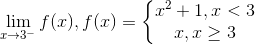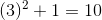# AP Calculus BC : Calculating Limits with Algebra (including one-sided limits)

## Example Questions

### Example Question #1 : Calculating Limits With Algebra (Including One Sided Limits)

Evaluate the following limit:The limit does not existExplanation:

The limit we are given is one sided, meaning we are approaching our x value from one side; in this case, the negative sign exponent indicates that we are approaching 3 from the left side, or using values slightly less than three on approach.

This corresponds to the part of the piecewise function for values less than 3. When we substitute our x value being approached, we get### All AP Calculus BC Resources